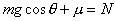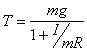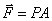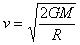Teaching Physics with the Physics Suite Edward F. Redish

Well-formed formulas

Each of the mathematical expressions below was given by a student on his or her way to the solution of an exam problem. Assume each of the symbols stands for what we use them for in this class (as indicated in each line). If you believe the equation given could possibly be a correct physical equation, indicate Y . If you think that it could not possibly be a correct physical equation, indicate N and give a brief explanation of why you think so.

 (a)(mass, gravitational field strength, coefficient of friction, normal force) (b)(tension, mass, gravitational field strength, moment of inertia, radius) (c)(force, pressure, area) (d)(velocity, universal gravitational constant, mass, radius)

Not finding what you wanted? Check the Site Map for more information.